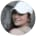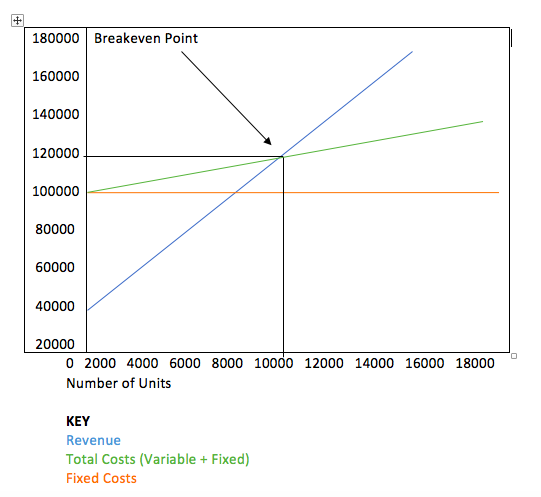AnnaMarie Houlis4.87k
Journalist & travel blogger

Starting a new business, selling a new product or offering a new service is no easy feat. It's not necessarily clear from the start just how profitable your new business will be, or how just how successful your new product or service will end up. What you need to help you determine when you'll break even and start earning is a breakeven analysis.

A breakeven analysis can help your company determine when exactly it'll become profitable. Here's what to know about what a breakeven analysis formula is, how to read one, when to use one and how to create your own breakeven analysis.

## What is the breakeven analysis formula?

A breakeven analysis formula is a mathematical formula that can help you determine when your company will break even and, as such, when and how to become profitable.

More specifically, "the breakeven analysis (BEA) is a useful tool to study the relation between fixed costs and variable costs and revenue," according to Tools Hero. "It’s inextricably linked to the breakeven point (BEP), which indicates at what moment an investment will start generating a positive return. It can be graphically represented or calculated with a simple mathematical calculation. A breakeven analysis calculates the size of the production at a certain (selling) price that is necessary to cover all the costs that have been incurred."

So what's a breakeven point? It determines when exactly a project will be profitable.

"Break-even analysis is useful in the determination of the level of production or a targeted desired sales mix," according to Investopedia. "The study is for management’s use only, as the metric and calculations are not necessary for external sources such as investors, regulators or financial institutions. This type of analysis depends on a calculation of the breakeven point."

That said, investors may use a breakeven analysis to determine the price at which they'll break even on a trade or investment. So the forumla is useful when trading in or creating strategies to buy options or fixed-income security products.

## What are the components of a breakeven analysis?

There are two main components of a breakeven analysis.

### 1. Fixed Costs

The fixed costs, also known as the overhead, are the costs that occur when a company makes a decision to start an economic activity. They relate to the level, not the quantity, of production. These costs include the depreciation of materials, taxes, interest and other overhead costs like labor, energy and more.

### 2. Variable Costs

Variable costs, unlike fixed costs, are those that do indeed change in direct relation to the production volume. These might be selling costs, production costs, labor costs, fuel costs and other variable costs for things like raw and auxiliary materials.

Some other terms you should know and understand include the following:

• The sales price per unit refers to the selling price (unit selling price) per unit.
• Variable cost per unit refers to the variable costs that are incurred in order to create a unit.
• Revenue refers to the price for which you're selling the product, sans the variable costs.

## How do you make breakeven calculations?

So you're probably wondering, how do you calculate the breakeven point? or how do you read a breakeven analysis chart?.

It's important to note that there are two basic formulas that are used in order to determine a business’s breakeven point. While one is based on the number of units of a product sold, the other is based on points in sales dollars.

### Breakeven Point Calculation Based on Product Units Sold

To calculate a breakeven point that's based on units of products sold, simply divide the fixed costs by the revenue per unit sans the variable cost per unit. Again, the fixed costs are those that do not change regardless of how many units are sold. And your revenue refers to the price for which you’re selling the product (without all the variable costs, such as labor and raw materials).

"Since the price per unit minus the variable costs of production is the definition of the contribution margin per unit, you can simply rephrase the equation by dividing the fixed costs by the contribution margin," according to My Accounting Course. This computes the total number of units that must be sold in order for the company to generate enough revenues to cover all of its expenses."

You read the breakeven point simply. Breakeven Point = fixed costs / ( selling price – variable costs )

### Breakeven Point Calculation Based on Sales Dollars

To calculate a breakeven point based on sales dollars, divide the fixed costs by the contribution margin. You can determine the contribution margin by subtracting the variable costs from the price of the product. And that amount is used to cover any and all fixed costs.

Here's a real-world example from Investopedia:

"Suppose an options trader buys a 50-strike call for \$1.00 premium when the underlying is trading at \$46. A break-even analysis will show that the price of the underlying must reach \$51 before they break-even on the trade. While the call will be in-the-money (ITM) at any price trading above \$50, the trader will still need to recoup the option premium of \$1 which they originally paid to buy the option."

## When would you use a breakeven analysis?

The breakeven analysis comes in handy for companies when they're deciding whether or not they should start producing and selling a product. That's because it's a handy tool to calculate the breakeven point, which is also a critical point that's the turnover at which the company's total revenue would finally equal the total costs. If the turnover is lower than the total costs, however, the company experiences a loss.

## What's an example of a breakeven analysis chart?

There are tons of examples of breakeven analysis charts online. To help you get a better understanding of what a breakeven analysis chart looks like, here's an example.